Do you like the article?
Share it with others -

Use new possibilities of MetaTrader 5

# Graphical Interfaces X: Text selection in the Multiline Text box (build 13)

27 June 2017, 13:18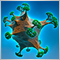0
10 230

### Introduction

The first article Graphical Interfaces I: Preparation of the Library Structure (Chapter 1) considers in detail what this library is for. A complete list of links to the articles of the first part is at the end of each chapter. There, you can also download a complete version of the library at the current stage of development. Files must be placed under the same directories as they are located in the archive.

In order to fully utilize the Multiline text box considered in the articles listed below, it is necessary to implement text selection, because deleting text one character at a time is inconvenient.

Selecting text using the key combinations and deleting the selected text will look exactly the same as in any other text editor. In addition, we will continue to optimize the code and prepare the classes to move on to the final process of the second stage of the library's evolution, where all controls will be rendered as separate images (canvases).

The final version of this control will be presented here. Any subsequent modification will be made only if a more efficient solution regarding a certain algorithm is found.

### Tracking the pressing of the Shift key

First of all, let us add the CKeys::KeyShiftState() method for determining the current state of the Shift key to the previously considered CKeys class, which is designed for working with the keyboard. This key will be used in various combinations for selecting text. The listing below shows the code of this simple method. The Shift key is considered to be pressed, if the ::TerminalInfoInteger() function with the TERMINAL_KEYSTATE_SHIFT identifier returns a value less than zero.

```//+------------------------------------------------------------------+
//| Class for working with the keyboard                              |
//+------------------------------------------------------------------+
class CKeys
{
public:
//--- Returns the state of the Shift key
bool              KeyShiftState(void);
};
//+------------------------------------------------------------------+
//| Returns the state of the Shift key                               |
//+------------------------------------------------------------------+
bool CKeys::KeyShiftState(void)
{
return(::TerminalInfoInteger(TERMINAL_KEYSTATE_SHIFT)<0);
}
```

### Key combinations for selecting text

Let us consider all key combinations for selecting text, which will be implemented in the Text box. We will start with a combination of two keys.

• The 'Shift + Left' and 'Shift + Right' combinations shift the text cursor to the left and right by one character, respectively. The text is highlighted with a different color of background and character (also customizable by user):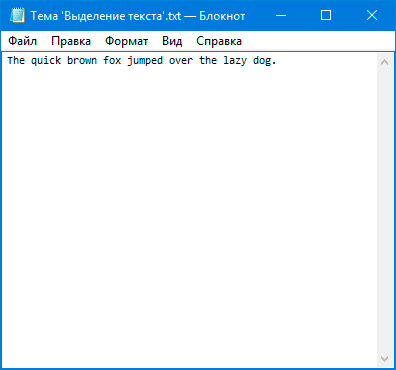Fig. 1 Selection of text with a shift to the left and right by one character.

• The 'Shift + Home' and 'Shift + End' combinations shift the text cursor to the beginning and end of the line, selecting all characters from the starting position of the cursor.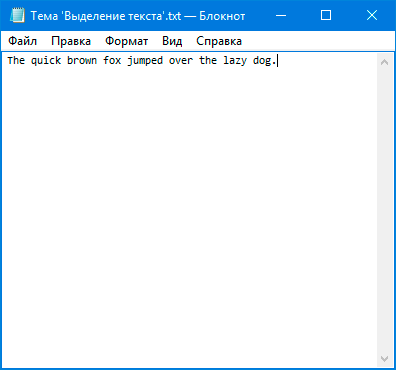Fig. 2. Selection of text with the cursor shifted from the starting position to the beginning and end of the line.

• The 'Shift + Up' and 'Shift + Down' combinations shift the text cursor up and down by one line, respectively. The text is selected from the cursor to the beginning of the initial line and from the end of the final line up to the cursor. The behavior is symmetric for the 'Shift + Down' combination. If there are more lines between the initial and final selected lines, their text is selected completely.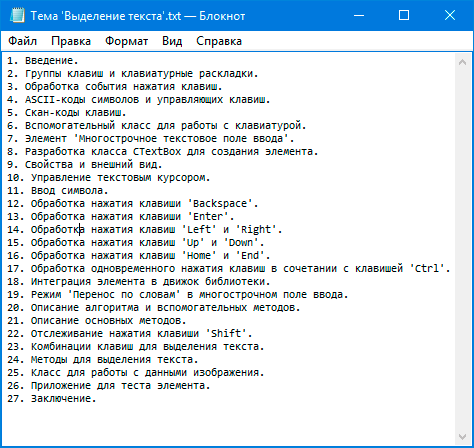Fig. 3. Selection of text with a shift by one line up and down.

Combinations of three keys are sometimes used for selecting text. For example, when it is necessary to quickly select several words on one line, a character-by-character selection will be too tedious. And if it is also necessary to select text that consists of multiple lines, even a line-by-line selection will not be convenient.

In combinations of three keys, Ctrl is used in addition to Shift. Let us consider all such combinations, which will be implemented in this article:

• The 'Ctrl + Shift + Left' and 'Ctrl + Shift + Right' combinations are intended to select text in whole words to the left and right of the current position of the text cursor, respectively: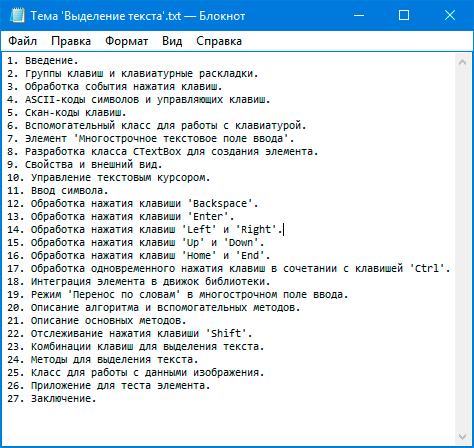Fig. 4. Selection of text with a shift to the left and right by one word.

• The 'Ctrl + Shift + Home' and 'Ctrl + Shift + End' combinations allow selecting all text up to the beginning of the first and the end of the last lines starting from the current position of the text cursor: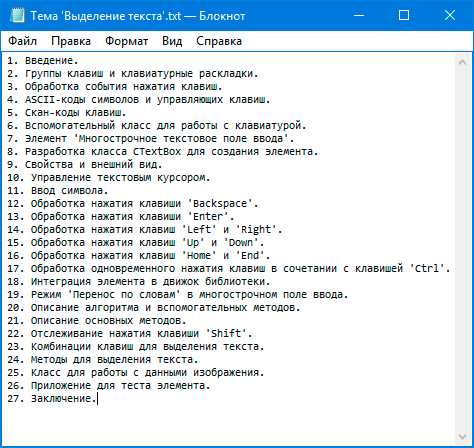Fig. 5. Selection of text with the cursor shifted to the beginning and end of the document.

The next section considers the methods used in text selection.

### Methods for selecting text

By default, the selected text is displayed with white characters on a blue background. If necessary, the CTextBox:: SelectedBackColor() and CTextBox:: SelectedTextColor() methods can be used to change the colors.

```class CTextBox : public CElement
{
private:
//--- Background and character colors of the selected text
color             m_selected_back_color;
color             m_selected_text_color;
//---
private:
//--- Background and character colors of the selected text
void              SelectedBackColor(const color clr)        { m_selected_back_color=clr;       }
void              SelectedTextColor(const color clr)        { m_selected_text_color=clr;       }
};
//+------------------------------------------------------------------+
//| Constructor                                                      |
//+------------------------------------------------------------------+
CTextBox::CTextBox(void) : m_selected_text_color(clrWhite),
m_selected_back_color(C'51,153,255')
{
//...
}
```

In order to select text, fields and methods for designating the initial and final indexes of the lines and characters of the selected text are required. In addition, a method will be needed for resetting those values when the selection is canceled.

Every time a key combination for selecting text is pressed, the CTextBox::SetStartSelectedTextIndexes() method will be called before shifting the text cursor. It sets the initial line and character index values, where the text cursor is located. The values will be set only if it is the first call to the method after those values were last reset. The cursor is shifted after this method is called. Then the CTextBox::SetEndSelectedTextIndexes() method is called, which sets the final values of the line and character indexes (that is, the cursor position of the text cursor). If during the process of moving the text cursor in the text selection mode it turns out that the cursor is located right where it started, the values are reset by calling the CTextBox::ResetSelectedText() method. The values are also reset on any movement of the text cursor, deletion of the selected text or deactivation of the text box.

```class CTextBox : public CElement
{
private:
//--- The start and end indexes of lines and characters (of the selected text)
int               m_selected_line_from;
int               m_selected_line_to;
int               m_selected_symbol_from;
int               m_selected_symbol_to;
//---
private:
//--- Sets the (1) start and (2) end indexes for text selection
void              SetStartSelectedTextIndexes(void);
void              SetEndSelectedTextIndexes(void);
//--- Reset the selected text
void              ResetSelectedText(void);
};
//+------------------------------------------------------------------+
//| Set the start indexes for selecting text                         |
//+------------------------------------------------------------------+
void CTextBox::SetStartSelectedTextIndexes(void)
{
//--- If the starting indexes for selecting text have not been set yet
if(m_selected_line_from==WRONG_VALUE)
{
m_selected_line_from   =(int)m_text_cursor_y_pos;
m_selected_symbol_from =(int)m_text_cursor_x_pos;
}
}
//+------------------------------------------------------------------+
//| Set the end indexes for selecting text                           |
//+------------------------------------------------------------------+
void CTextBox::SetEndSelectedTextIndexes(void)
{
//--- Set the end indexes for selecting text
m_selected_line_to   =(int)m_text_cursor_y_pos;
m_selected_symbol_to =(int)m_text_cursor_x_pos;
//--- If all indexes are the same, clear the selection
if(m_selected_line_from==m_selected_line_to && m_selected_symbol_from==m_selected_symbol_to)
ResetSelectedText();
}
//+------------------------------------------------------------------+
//| Reset the selected text                                          |
//+------------------------------------------------------------------+
void CTextBox::ResetSelectedText(void)
{
m_selected_line_from   =WRONG_VALUE;
m_selected_line_to     =WRONG_VALUE;
m_selected_symbol_from =WRONG_VALUE;
m_selected_symbol_to   =WRONG_VALUE;
}
```

Blocks of code previously used in methods for moving the cursor are now implemented as separate methods, as they will be repeatedly used in text selection methods. The same applies to the code for adjusting the scrollbars in cases when the text cursor goes beyond the visible area.

```class CTextBox : public CElement
{
private:
//--- Moving the text cursor to the left by one character
void              MoveTextCursorToLeft(void);
//--- Moving the text cursor to the right by one character
void              MoveTextCursorToRight(void);
//--- Moving the text cursor up by one character
void              MoveTextCursorToUp(void);
//--- Moving the text cursor down by one character
void              MoveTextCursorToDown(void);

void              CorrectingHorizontalScrollThumb(void);
void              CorrectingVerticalScrollThumb(void);
};
```

All methods for handling the pressing the combinations of keys (one of which is Shift) contain virtually the same code, except for calling the method for moving the text cursor. Therefore, it is reasonable to create an additional method that can simply be passed the direction to move the text cursor. The ENUM_MOVE_TEXT_CURSOR enumeration with several identifiers (see the listing below) has been added to the Enums.mqh file. They can be used to indicate where the text cursor needs to be moved:

• TO_NEXT_LEFT_SYMBOL — one character to the left.
• TO_NEXT_RIGHT_SYMBOL — one character to the right.
• TO_NEXT_LEFT_WORD — one word to the left.
• TO_NEXT_RIGHT_WORD — one word to the right.
• TO_NEXT_UP_LINE — one line up.
• TO_NEXT_DOWN_LINE — one line down.
• TO_BEGIN_LINE — to the beginning of the current line.
• TO_END_LINE — to the end of the current line.
• TO_BEGIN_FIRST_LINE — to the beginning of the first line.
• TO_END_LAST_LINE — to the end of the last line.

```//+------------------------------------------------------------------+
//| Enumeration for the direction to move the text cursor            |
//+------------------------------------------------------------------+
enum ENUM_MOVE_TEXT_CURSOR
{
TO_NEXT_LEFT_SYMBOL  =0,
TO_NEXT_RIGHT_SYMBOL =1,
TO_NEXT_LEFT_WORD    =2,
TO_NEXT_RIGHT_WORD   =3,
TO_NEXT_UP_LINE      =4,
TO_NEXT_DOWN_LINE    =5,
TO_BEGIN_LINE        =6,
TO_END_LINE          =7,
TO_BEGIN_FIRST_LINE  =8,
TO_END_LAST_LINE     =9
};
```

We can now create a common general method for moving the text cursor — CTextBox::MoveTextCursor(), where passing one of the identifiers from the list above is sufficient. The same method will now be used in virtually all methods that handle the keypress events in the CTextBox control.

```class CTextBox : public CElement
{
private:
//--- Moving the text cursor in the specified direction
void              MoveTextCursor(const ENUM_MOVE_TEXT_CURSOR direction);
};
//+------------------------------------------------------------------+
//| Moving the text cursor in the specified direction                |
//+------------------------------------------------------------------+
void CTextBox::MoveTextCursor(const ENUM_MOVE_TEXT_CURSOR direction)
{
switch(direction)
{
//--- Move the cursor one character to the left
case TO_NEXT_LEFT_SYMBOL  : MoveTextCursorToLeft();        break;
//--- Move the cursor one character to the right
case TO_NEXT_RIGHT_SYMBOL : MoveTextCursorToRight();       break;
//--- Move the cursor one word to the left
case TO_NEXT_LEFT_WORD    : MoveTextCursorToLeft(true);    break;
//--- Move the cursor one word to the right
case TO_NEXT_RIGHT_WORD   : MoveTextCursorToRight(true);   break;
//--- Move the cursor one line up
case TO_NEXT_UP_LINE      : MoveTextCursorToUp();          break;
//--- Move the cursor one line down
case TO_NEXT_DOWN_LINE    : MoveTextCursorToDown();        break;
//--- Move the cursor to the beginning of the current line
case TO_BEGIN_LINE : SetTextCursor(0,m_text_cursor_y_pos); break;
//--- Move the cursor to the end of the current line
case TO_END_LINE :
{
//--- Get the number of characters in the current line
uint symbols_total=::ArraySize(m_lines[m_text_cursor_y_pos].m_symbol);
//--- Move the cursor
SetTextCursor(symbols_total,m_text_cursor_y_pos);
break;
}
//--- Move the cursor to the beginning of the first line
case TO_BEGIN_FIRST_LINE : SetTextCursor(0,0); break;
//--- Move the cursor to the end of the last line
case TO_END_LAST_LINE :
{
//--- Get the number of lines and characters in the last line
uint lines_total   =::ArraySize(m_lines);
uint symbols_total =::ArraySize(m_lines[lines_total-1].m_symbol);
//--- Move the cursor
SetTextCursor(symbols_total,lines_total-1);
break;
}
}
}
```

The code in this file can be significantly reduced, because the handler methods of the text cursor movement and the text selection events have a lot of repeated code blocks.

Example of a repeated code block in the methods for moving the text cursor:

```//+------------------------------------------------------------------+
//| Handling the pressing of the Left key                            |
//+------------------------------------------------------------------+
bool CTextBox::OnPressedKeyLeft(const long key_code)
{
//--- Leave, if (1) it is not the Left key or (2) the Ctrl key is pressed or (3) the Shift key is pressed
if(key_code!=KEY_LEFT || m_keys.KeyCtrlState() || m_keys.KeyShiftState())
return(false);
//--- Reset the selection
ResetSelectedText();
//--- Shift the text cursor to the left by one character
MoveTextCursor(TO_NEXT_LEFT_SYMBOL);
CorrectingHorizontalScrollThumb();
CorrectingVerticalScrollThumb();
//--- Update the text in the text box
DrawTextAndCursor(true);
//--- Send a message about it
::EventChartCustom(m_chart_id,ON_MOVE_TEXT_CURSOR,CElementBase::Id(),CElementBase::Index(),TextCursorInfo());
return(true);
}
```

Example of a repeated code block in the methods for selecting the text:

```//+------------------------------------------------------------------+
//| Handling the pressing of the Shift + Left keys                   |
//+------------------------------------------------------------------+
bool CTextBox::OnPressedKeyShiftAndLeft(const long key_code)
{
//--- Leave, if (1) it is not the Left key or (2) the Ctrl key is pressed or (3) the Shift key is not pressed
if(key_code!=KEY_LEFT || m_keys.KeyCtrlState() || !m_keys.KeyShiftState())
return(false);
//--- Set the start indexes for selecting text
SetStartSelectedTextIndexes();
//--- Shift the text cursor to the left by one character
MoveTextCursor(TO_NEXT_LEFT_SYMBOL);
//--- Set the end indexes for selecting text
SetEndSelectedTextIndexes();
CorrectingHorizontalScrollThumb();
CorrectingVerticalScrollThumb();
//--- Update the text in the text box
DrawTextAndCursor(true);
//--- Send a message about it
::EventChartCustom(m_chart_id,ON_MOVE_TEXT_CURSOR,CElementBase::Id(),CElementBase::Index(),TextCursorInfo());
return(true);
}

```

Let us implement one more additional (overloaded) method CTextBox::MoveTextCursor(). The method will need to be passed the identifier with the movement direction, as well as the flag indicating (1) if it is a movement of the text cursor or (2) a text selection.

```class CTextBox : public CElement
{
private:
//--- Moving the text cursor in the specified direction
void              MoveTextCursor(const ENUM_MOVE_TEXT_CURSOR direction,const bool with_highlighted_text);
};
//+------------------------------------------------------------------+
//| Moving the text cursor in the specified direction and            |
//| with a condition                                                 |
//+------------------------------------------------------------------+
void CTextBox::MoveTextCursor(const ENUM_MOVE_TEXT_CURSOR direction,const bool with_highlighted_text)
{
//--- If it is only text cursor movement
if(!with_highlighted_text)
{
//--- Reset the selection
ResetSelectedText();
//--- Move the cursor to the beginning of the first line
MoveTextCursor(direction);
}
//--- If text selection is enabled
else
{
//--- Set the start indexes for selecting text
SetStartSelectedTextIndexes();
//--- Shift the text cursor to the left by one character
MoveTextCursor(direction);
//--- Set the end indexes for selecting text
SetEndSelectedTextIndexes();
}
CorrectingHorizontalScrollThumb();
CorrectingVerticalScrollThumb();
//--- Update the text in the text box
DrawTextAndCursor(true);
//--- Send a message about it
::EventChartCustom(m_chart_id,ON_MOVE_TEXT_CURSOR,CElementBase::Id(),CElementBase::Index(),TextCursorInfo());
}

```

Methods for handling the key combinations for selecting text are shown below. Their code is almost identical (they only differ in parameters). Therefore, you can study the code in the files attached to the article:

```class CTextBox : public CElement
{
private:
//--- Handling the pressing of the Shift + Left keys
bool              OnPressedKeyShiftAndLeft(const long key_code);
//--- Handling the pressing of the Shift + Right keys
bool              OnPressedKeyShiftAndRight(const long key_code);
//--- Handling the pressing of the Shift + Up keys
bool              OnPressedKeyShiftAndUp(const long key_code);
//--- Handling the pressing of the Shift + Down keys
bool              OnPressedKeyShiftAndDown(const long key_code);
//--- Handling the pressing of the Shift + Home keys
bool              OnPressedKeyShiftAndHome(const long key_code);
//--- Handling the pressing of the Shift + End keys
bool              OnPressedKeyShiftAndEnd(const long key_code);

//--- Handling the pressing of the Ctrl + Shift + Left keys
bool              OnPressedKeyCtrlShiftAndLeft(const long key_code);
//--- Handling the pressing of the Ctrl + Shift + Right keys
bool              OnPressedKeyCtrlShiftAndRight(const long key_code);
//--- Handling the pressing of the Ctrl + Shift + Home keys
bool              OnPressedKeyCtrlShiftAndHome(const long key_code);
//--- Handling the pressing of the Ctrl + Shift + End keys
bool              OnPressedKeyCtrlShiftAndEnd(const long key_code);
};

```

So far the text was applied to canvas in whole lines. But since the selected characters and the background under them change color, the text must be output character-wise. To do this, let us make some small changes to CTextBox::TextOut() method.

It will also require an additional CTextBox::CheckSelectedText() method for checking the selected characters. We already know that during the selection of text, the indexes of the initial and final lines and characters of the text cursor are stored. Therefore, it is easy to determine if a character in a line is selected or not by iterating over the characters in a loop. The logic is simple:

1. If the initial index of the line is lower than the final one, the character is selected:
• If this is the final line and the character is to the right of the final selected one
• If this is the initial line and the character is to the left of the initial selected one
• All characters are selected on the intermediate lines
2. If the initial index of the line is higher than the final one, the character is selected:
• If this is the final line and the character is to the left of the final selected one
• If this is the initial line and the character is to the right of the initial selected one
• All characters are selected on the intermediate lines
3. If the text is selected on one line only, then a character is selected if it is within the designated range between the initial and final character indexes.

```class CTextBox : public CElement
{
private:
//--- Check for presence of selected text
bool              CheckSelectedText(const uint line_index,const uint symbol_index);
};
//+------------------------------------------------------------------+
//| Check for presence of selected text                              |
//+------------------------------------------------------------------+
bool CTextBox::CheckSelectedText(const uint line_index,const uint symbol_index)
{
bool is_selected_text=false;
//--- Leave, if there is no selected text
if(m_selected_line_from==WRONG_VALUE)
return(false);
//--- If the initial index is on the line below
if(m_selected_line_from>m_selected_line_to)
{
//--- The final line and the character to the right of the final selected one
if((int)line_index==m_selected_line_to && (int)symbol_index>=m_selected_symbol_to)
{ is_selected_text=true; }
//--- The initial line and the character to the left of the initial selected one
else if((int)line_index==m_selected_line_from && (int)symbol_index<m_selected_symbol_from)
{ is_selected_text=true; }
//--- Intermediate line (all characters are selected)
else if((int)line_index>m_selected_line_to && (int)line_index<m_selected_line_from)
{ is_selected_text=true; }
}
//--- If the initial index is on the line above
else if(m_selected_line_from<m_selected_line_to)
{
//--- The final line and the character to the left of the final selected one
if((int)line_index==m_selected_line_to && (int)symbol_index<m_selected_symbol_to)
{ is_selected_text=true; }
//--- The initial line and the character to the right of the initial selected one
else if((int)line_index==m_selected_line_from && (int)symbol_index>=m_selected_symbol_from)
{ is_selected_text=true; }
//--- Intermediate line (all characters are selected)
else if((int)line_index<m_selected_line_to && (int)line_index>m_selected_line_from)
{ is_selected_text=true; }
}
//--- If the initial and final indexes are on the same line
else
{
//--- Find the checked line
if((int)line_index>=m_selected_line_to && (int)line_index<=m_selected_line_from)
{
//--- If the cursor is shifted to the right and the character is within the selected range
if(m_selected_symbol_from>m_selected_symbol_to)
{
if((int)symbol_index>=m_selected_symbol_to && (int)symbol_index<m_selected_symbol_from)
is_selected_text=true;
}
//--- If the cursor is shifted to the left and the character is within the selected range
else
{
if((int)symbol_index>=m_selected_symbol_from && (int)symbol_index<m_selected_symbol_to)
is_selected_text=true;
}
}
}
//--- Return the result
return(is_selected_text);
}
```

In the CTextBox::TextOut() method designed for outputting text, it is necessary to add an internal cycle with an iteration over the line's characters instead of outputting the entire line. It determines if the checked character is selected. In case the character is selected, its color is determined and a filled rectangle is drawn under the character. After all this, the character itself is output

```class CTextBox : public CElement
{
private:
//--- Output text to canvas
void              TextOut(void);
};
//+------------------------------------------------------------------+
//| Output text to canvas                                            |
//+------------------------------------------------------------------+
void CTextBox::TextOut(void)
{
//--- Clear canvas
m_canvas.Erase(AreaColorCurrent());
//--- Get the size of the lines array
uint lines_total=::ArraySize(m_lines);
//--- Correction in case the size was exceeded
m_text_cursor_y_pos=(m_text_cursor_y_pos>=lines_total)? lines_total-1 : m_text_cursor_y_pos;
//--- Get the size of the array of characters
uint symbols_total=::ArraySize(m_lines[m_text_cursor_y_pos].m_symbol);
//--- If multiline mode is enabled or if the number of characters is greater than zero
if(m_multi_line_mode || symbols_total>0)
{
//--- Get the line width
int line_width=(int)LineWidth(m_text_cursor_x_pos,m_text_cursor_y_pos);
//--- Get the line height and iterate over all lines in a loop
int line_height=(int)LineHeight();
for(uint i=0; i<lines_total; i++)
{
//--- Get the coordinates for the text
int x=m_text_x_offset;
int y=m_text_y_offset+((int)i*line_height);
//--- Get the string size
uint string_length=::ArraySize(m_lines[i].m_symbol);
//--- Draw the text
for(uint s=0; s<string_length; s++)
{
uint text_color=TextColorCurrent();
//--- If there is a selected text, determine its color and the background color of the current character
if(CheckSelectedText(i,s))
{
//--- Color of the selected text
text_color=::ColorToARGB(m_selected_text_color);
//--- Calculate the coordinates for drawing the background
int x2=x+m_lines[i].m_width[s];
int y2=y+line_height-1;
//--- Draw the background color of the character
m_canvas.FillRectangle(x,y,x2,y2,::ColorToARGB(m_selected_back_color));
}
//--- Draw the character
m_canvas.TextOut(x,y,m_lines[i].m_symbol[s],text_color,TA_LEFT);
//--- X coordinate for the next character
x+=m_lines[i].m_width[s];
}
}
}
//--- If the multiline mode is disabled and there is no character, the default text will be displayed
else
{
//--- Draw text, if specified
if(m_default_text!="")
m_canvas.TextOut(m_area_x_size/2,m_area_y_size/2,m_default_text,::ColorToARGB(m_default_text_color),TA_CENTER|TA_VCENTER);
}
}
```

Methods for selecting text have been implemented, and this is how it looks in the finished application: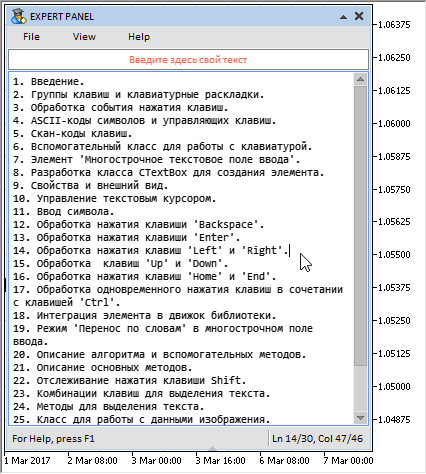Fig. 6. Demonstration of text selection in the implemented text box of the MQL application.

### Methods for deleting the selected text

Now let us consider the methods for deleting the selected text. Here, it is important to note that different methods will be applied when deleting selected text, depending on whether it is selected on one or multiple lines.

The CTextBox::DeleteTextOnOneLine() method will be called for deleting text selected on a single line. The number of characters to be deleted is determined at the beginning of the method. Then, if the initial index of the selected text's character is on the right, the characters are shifted right starting from this initial position by the number of characters to be deleted. After that, the array of line's characters is decreased by the same amount.

In the cases where the initial index of the selected text's character is on the left, the text cursor also needs to be shifted to the right by the number of characters to be deleted.

```class CTextBox : public CElement
{
private:
//--- Deletes text selected on one line
void              DeleteTextOnOneLine(void);
};
//+------------------------------------------------------------------+
//| Deletes text selected on one line                                |
//+------------------------------------------------------------------+
void CTextBox::DeleteTextOnOneLine(void)
{
int symbols_total     =::ArraySize(m_lines[m_text_cursor_y_pos].m_symbol);
int symbols_to_delete =::fabs(m_selected_symbol_from-m_selected_symbol_to);
//--- If the initial index of the character is on the right
if(m_selected_symbol_to<m_selected_symbol_from)
{
//--- Shift the characters to the freed area in the current line
MoveSymbols(m_text_cursor_y_pos,m_selected_symbol_from,m_selected_symbol_to);
}
//--- If the initial index of the character is on the left
else
{
//--- Shift the text cursor to the left by the number of characters to be deleted
m_text_cursor_x_pos-=symbols_to_delete;
//--- Shift the characters to the freed area in the current line
MoveSymbols(m_text_cursor_y_pos,m_selected_symbol_to,m_selected_symbol_from);
}
//--- Decrease the array size of the current line by the number of extracted characters
ArraysResize(m_text_cursor_y_pos,symbols_total-symbols_to_delete);
}

```

The CTextBox::DeleteTextOnMultipleLines() method will be used for deleting multiple lines of the selected text. The algorithm is more complicated here. First, it is necessary to determine:

• The total number of characters on the initial and final lines
• The number of intermediate lines of the selected text (except the initial and final lines)
• The number of characters to be deleted on the initial and final lines.

The sequence of further actions is given below. Depending on the direction the text is selected (up or down), the initial and final indexes will be passed to other methods.

• The characters to be moved from one line to another, which will remain after the deletion, are copied to a temporary dynamic array.
• The receiver array (line) is resized.
• Data are added to the arrays of the receiver line structure.
• The lines are shifted by the number of deleted lines.
• The array of lines is resized (decreased by the number of deleted lines).
• In case the initial line is above the final line (text selection is downwards), the text cursor is shifted to the initial indexes (line and character) of the selected text.

```class CTextBox : public CElement
{
private:
//--- Deletes text selected on multiple lines
void              DeleteTextOnMultipleLines(void);
};
//+------------------------------------------------------------------+
//| Deletes text selected on multiple lines                          |
//+------------------------------------------------------------------+
void CTextBox::DeleteTextOnMultipleLines(void)
{
//--- The total number of characters on the initial and final lines
uint symbols_total_line_from =::ArraySize(m_lines[m_selected_line_from].m_symbol);
uint symbols_total_line_to   =::ArraySize(m_lines[m_selected_line_to].m_symbol);
//--- The number of intermediate lines to be deleted
uint lines_to_delete =::fabs(m_selected_line_from-m_selected_line_to);
//--- The number of characters to be deleted on the initial and final lines
uint symbols_to_delete_in_line_from =::fabs(symbols_total_line_from-m_selected_symbol_from);
uint symbols_to_delete_in_line_to   =::fabs(symbols_total_line_to-m_selected_symbol_to);
//--- If the initial line is below the final line
if(m_selected_line_from>m_selected_line_to)
{
//--- Copy the characters to be moved into the array
string array[];
CopyWrapSymbols(m_selected_line_from,m_selected_symbol_from,symbols_to_delete_in_line_from,array);
uint new_size=m_selected_symbol_to+symbols_to_delete_in_line_from;
ArraysResize(m_selected_line_to,new_size);
PasteWrapSymbols(m_selected_line_to,m_selected_symbol_to,array);
//--- Get the size of the lines array
uint lines_total=::ArraySize(m_lines);
//--- Shift lines up by the number of lines to be deleted
MoveLines(m_selected_line_to+1,lines_total-lines_to_delete,lines_to_delete,false);
//--- Resize the lines array
::ArrayResize(m_lines,lines_total-lines_to_delete);
}
//--- If the initial line is above the final line
else
{
//--- Copy the characters to be moved into the array
string array[];
CopyWrapSymbols(m_selected_line_to,m_selected_symbol_to,symbols_to_delete_in_line_to,array);
uint new_size=m_selected_symbol_from+symbols_to_delete_in_line_to;
ArraysResize(m_selected_line_from,new_size);
PasteWrapSymbols(m_selected_line_from,m_selected_symbol_from,array);
//--- Get the size of the lines array
uint lines_total=::ArraySize(m_lines);
//--- Shift lines up by the number of lines to be deleted
MoveLines(m_selected_line_from+1,lines_total-lines_to_delete,lines_to_delete,false);
//--- Resize the lines array
::ArrayResize(m_lines,lines_total-lines_to_delete);
//--- Move the cursor to the initial position in the selection
SetTextCursor(m_selected_symbol_from,m_selected_line_from);
}
}
```

Which of the above methods to call is determined in the main method for deleting text — CTextBox::DeleteSelectedText(). Once the selected text is deleted, the values of the initial and final indexes are reset. After that, it is necessary to recalculate the text box dimensions, as the number of lines might have changed. Also, the maximum width of the line could have changed, which is used in calculation of the text box. At the end, the method sends a message that the text cursor has moved. The method returns true if the text was selected and removed. If it turns out that there is no selected text at the time the method is called, it returns false

```class CTextBox : public CElement
{
private:
//--- Deletes the selected text
void              DeleteSelectedText(void);
};
//+------------------------------------------------------------------+
//| Deletes the selected text                                        |
//+------------------------------------------------------------------+
bool CTextBox::DeleteSelectedText(void)
{
//--- Leave, if no text is selected
if(m_selected_line_from==WRONG_VALUE)
return(false);
//--- If characters are deleted from one line
if(m_selected_line_from==m_selected_line_to)
DeleteTextOnOneLine();
//--- If characters are deleted from multiple lines
else
DeleteTextOnMultipleLines();
//--- Reset the selected text
ResetSelectedText();
//--- Calculate the size of the text box
CalculateTextBoxSize();
//--- Set the new size to the text box
ChangeTextBoxSize();
CorrectingHorizontalScrollThumb();
CorrectingVerticalScrollThumb();
//--- Update the text in the text box
DrawTextAndCursor(true);
//--- Send a message about it
::EventChartCustom(m_chart_id,ON_MOVE_TEXT_CURSOR,CElementBase::Id(),CElementBase::Index(),TextCursorInfo());
return(true);
}
```

The CTextBox::DeleteSelectedText() method is called not only when pressing the Backspace key, but also: (1) when entering a new character and (2) when pressing the Enter key. In these cases, the text is deleted first, and then the action corresponding to the pressed key is performed.

This is how it looks in the finished application: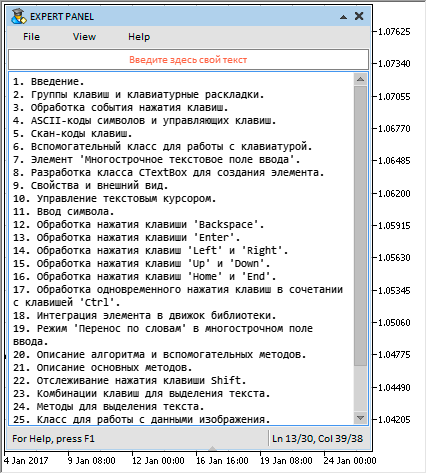Fig. 7. Demonstration of deleting the selected text.

### Class for working with image data

As a supplement to this article, let us consider a new class (CImage) for working with image data. It will be repeatedly used in many classes of the library's controls, which need to draw an image. The class is contained in the Objects.mqh file.

Class properties:
• array of image pixels;
• image width;
• image height;
• path to the image file.

```//+------------------------------------------------------------------+
//| Class for storing the image data                                 |
//+------------------------------------------------------------------+
class CImage
{
protected:
uint              m_image_data[]; // Array of the image pixels
uint              m_image_width;  // Image width
uint              m_image_height; // Image height
string            m_bmp_path;     // Path to the image file
public:
//--- (1) Size of the data array, (2) set/return the data (pixel color)
uint              DataTotal(void)                              { return(::ArraySize(m_image_data)); }
uint              Data(const uint data_index)                  { return(m_image_data[data_index]);  }
void              Data(const uint data_index,const uint data)  { m_image_data[data_index]=data;     }
//--- Set/return the image width
void              Width(const uint width)                      { m_image_width=width;               }
uint              Width(void)                                  { return(m_image_width);             }
//--- Set/return the image height
void              Height(const uint height)                    { m_image_height=height;             }
uint              Height(void)                                 { return(m_image_height);            }
//--- Set/return the path to the image
void              BmpPath(const string bmp_file_path)          { m_bmp_path=bmp_file_path;          }
string            BmpPath(void)                                { return(m_bmp_path);                }

};
//+------------------------------------------------------------------+
//| Constructor                                                      |
//+------------------------------------------------------------------+
CImage::CImage(void) : m_image_width(0),
m_image_height(0),
m_bmp_path("")
{
}
//+------------------------------------------------------------------+
//| Destructor                                                       |
//+------------------------------------------------------------------+
CImage::~CImage(void)
{
}
```

The CImage::ReadImageData() method is intended for saving the image and its properties. This method reads the image at the specified path and stores its data.

```class CImage
{
public:
//--- Read and store the data of the passed image
};
//+------------------------------------------------------------------+
//| Store the passed image to an array                               |
//+------------------------------------------------------------------+
{
//--- Reset the last error
::ResetLastError();
//--- Store the path to the image
m_bmp_file_path=bmp_file_path;
//--- Read and store the image data
{
::Print(__FUNCTION__," > error: ",::GetLastError());
return(false);
}
//---
return(true);
}
```

It may sometimes be necessary to make a copy of an image of the same type (CImage). For this purpose, the CImage::CopyImageData() method has been implemented. At the beginning of the method, the receiver array size is set to that of the source array. Then the data are copied from the source array to the receiver array in a cycle.

```class CImage
{
public:
//--- Copies the data of the passed image
void              CopyImageData(CImage &array_source);
};
//+------------------------------------------------------------------+
//| Copies the data of the passed image                              |
//+------------------------------------------------------------------+
void CImage::CopyImageData(CImage &array_source)
{
//--- Get the sizes of the receiver array and the source array
uint data_total        =DataTotal();
uint source_data_total =::GetPointer(array_source).DataTotal();
::ArrayResize(m_image_data,source_data_total);
//--- Copy the data
for(uint i=0; i<source_data_total; i++)
m_image_data[i]=::GetPointer(array_source).Data(i);
}
```

Prior to this update, the CCanvasTable class used a structure for storing the image data. Now, with the introduction of the CImage class, the corresponding changes have been made.

### Conclusion

This article concludes the development of the Multiline Text box control. Its key feature is that there are no more restrictions on the number of entered characters and multiple lines can be typed, which was ever so lacking in the standard graphical object of the OBJ_EDIT type. In the next article, we will continue to develop the topic of "Controls in table cells", adding the ability to change values in table cells using the control discussed in that article. In addition, several controls will be switched to a new mode: they will be rendered, and not built from multiple standard graphical objects.

Currently, the general schematic of the library for creating graphical interfaces looks as shown below: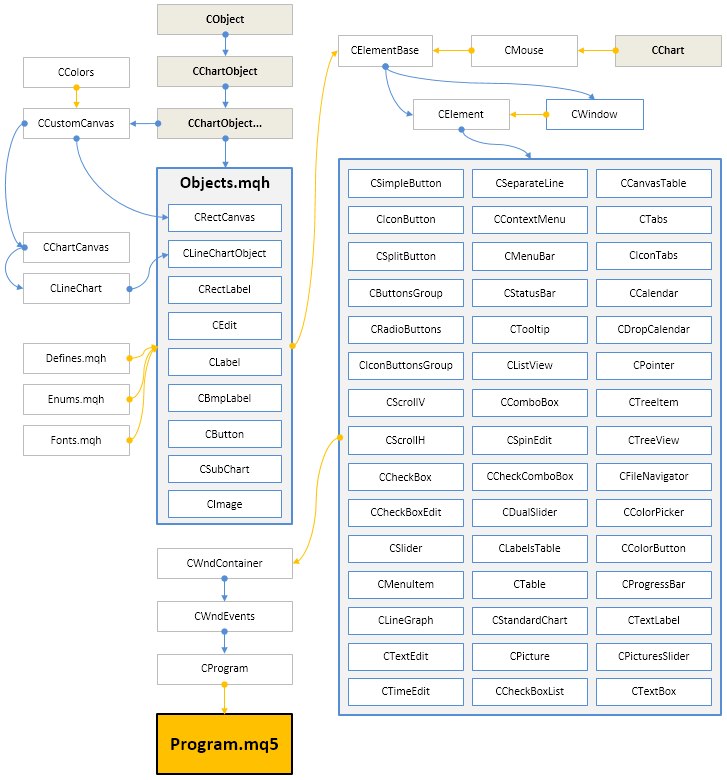Fig. 8. Library structure at the current stage of development.

Below are the latest version of the library and files for testing, which have been demonstrated in the article.

If you have questions on using the material presented in those files, you can refer to the detailed description of the library development in one of the articles of this series or ask your question in the comments of this article.

Translated from Russian by MetaQuotes Software Corp.
Original article: https://www.mql5.com/ru/articles/3197

Attached files |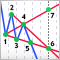Wolfe Waves

The graphical method proposed by Bill Wolfe allows to detect a pattern, based on which a moment and direction for entry can be found, and also it helps forecast the target which the price should reach, as well as the time of target reaching. The article describes how to create an indicator based on a ZigZag, that would search for Wolfe Waves, and a simple Expert Advisor trading based on this indicator.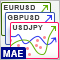Analyzing Balance/Equity graphs by symbols and EAs' ORDER_MAGIC

With the introduction of hedging, MetaTrader 5 provides an excellent opportunity to trade several Expert Advisors on a single trading account simultaneously. When one strategy is profitable, while the second one is loss-making, the profit graph may hang around zero. In this case, it is useful to build the Balance and Equity graphs for each trading strategy separately.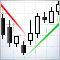An example of an indicator drawing Support and Resistance lines

The article provides an example of how to implement an indicator for drawing support and resistance lines based on formalized conditions. In addition to having a ready-to-use indicator, you will see how simple the indicator creation process is. You will also learn how to formulate conditions for drawing any desired line by changing the indicator code.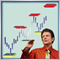DiNapoli trading system

The article describes the Fibo levels-based trading system developed by Joe DiNapoli. The idea behind the system and the main concepts are explained, as well as a simple indicator is provided as an example for more clarity.